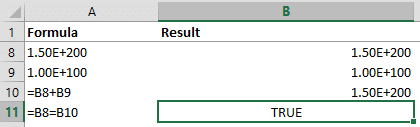As one of the most versatile calculators ever created, Excel is utilized to power innumerable business operations daily; however, can it ever fail to give you the correct results?

In the case of floating numbers, it can. The term floating point refers to the fact that there are no constant number of digits before or after the decimal point of a number. In other words, the decimal point itself can “float”.

Calculations may not show the correct results when dealing with high precision values. This is not a bug, but rather a design choice that affects every computing system to some degree.# Binary System

The main reason behind this behavior can be broken down to a fundamental design component of computer-based systems. Computer hardware and software communicate with one another using a binary system, consisting of values 1 and 0, as input and output data.

Therefore, the base-10 numerical system is also stored in binary format, which can cause issues with fractions. For example, the fraction of 2/10 is represented as 0.2 in the decimal number system, but identified by an infinitely repeating number 0.00110011001100110011 in the binary system. Data loss becomes inevitable when storing this number in a finite space. The data loss occurs after the 1E-15 point: more commonly known as the 15-digit limit in Excel.

For instance, looking at the example below, you would agree that cells C3 and C5 are equal. However, adding a value of 1 to the value of C3 changes the precision level (i.e. there are eleven 1’s in cell C4, while there are fifteen in cell C3). Ultimately, we get a completely different result in C5, which supposedly contains the inverse function.# Standard

Excel uses a modified version of the IEEE 754-1985 standards. This is a technical standard for floating-point computation, established in 1985 by the Institute of Electrical and Electronics Engineers (IEEE). The 754 revision became very popular and is the most widely used format for floating-point computation by both software libraries and hardware components (i.e. CPUs). According to Microsoft, it is used in all of today's PC-based microprocessors, including the Intel, Motorola, Sun, and MIPS processors.

# Limitations

## Maximum / Minimum

Excel only has a finite space to store data. This limitation affects the maximum and minimum numbers allowed by the software. Here are some examples:

 Smallest allowed negative number -2.2251e-308 Smallest allowed positive number 2.2251e-308 Largest allowed positive number 1e+308 Largest allowed negative number -1e+308 Largest allowed positive number via formula 1.79769e+308 Largest allowed negative number via formula -1.79769e+308

Source: https://support.office.com/en-us/article/excel-specifications-and-limits-1672b34d-7043-467e-8e27-269d656771c3

Excel returns the #NUM! error if a larger number is calculated. This behavior is called an overflow. Excel evaluates very small numbers as 0s, and this behavior is called as underflow. Both will result in data loss.

Excel does not support infinite numbers and provides a #DIV/0! error when a calculation results in an infinite number.

## Precision

Although Excel can display more than 15 digits, displayed values are not the actual values that are used in calculations. The IEEE standard upholds storage to only 15 significant digits, which can cause inconsistent results when working with very large or very small numbers.

### Very large numbers

In the example below, Excel finds the sum of B8 and B9 to be equal to the value of B8. The 15-digit limit causes Excel to evaluate the calculation with the 15 largest digits. This example would have required Excel to work with 100-digit precision to evaluate accurately.### Very small numbers

If we add the value 1 to 0.000123456789012345 (which has 18 significant digits but contains numeric values in its smallest 15 digits), the result should be 1.000123456789012345. However, Excel gives 1.00012345678901 because it ignores the digits following the 15th significant digit.## Repeating Binary Numbers

Storing repeating binary numbers as non-repeating decimal number can affect the final values in Excel. For example, the formula ‘=(73.1-73.2)+1’ evaluates to 0.899999999999991 when you format the decimal places to 15 digits.## Accuracy within VBA

Unlike Excel calculations, VBA can work with more than a single type of data. Excel uses 8-byte numbers, while VBA offers different data types that vary from 1 byte (Byte) to 16 bytes (Decimal), which can provide precision at the 28-digit level. You can choose a variable type based on your data storage, accuracy, and speed requirements.

Floating point calculations can be relatively challenging due to technical limitations. However, VBA and other mathematical methods offer workarounds to ensure that you don’t miss a decimal if you’re aware of the existing limitations.